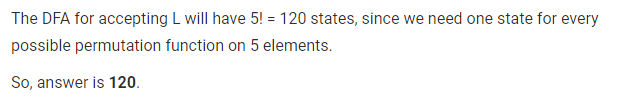# Gate CS-2019 Question Paper With Solutions

Q. 57 Let Σ be the set of all bijections from {1, …, 5} to {1, …, 5}, where id denotes the identity function, i.e. id(j) = j,∀j. Let ° denote composition on functions. For a string x = x1x2 … xn ∈ Σn, n ≥ 0, let π(x) = x1°x2° … °xn.

Consider the language L = {x ∈ Σ* ⏐ π(x) = id}. The minimum number of states in any DFA accepting L is _________ .

Note: This was Numerical Type question.

(A) 120

(B) 125

(C) 210

(D) None of these